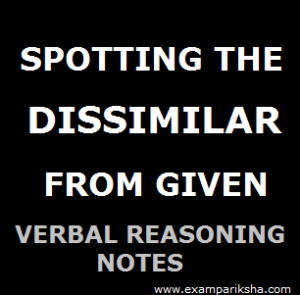In this type of question in reasoning section, the candidate has to choose one of the alternatives. Out of the given alternatives, only one is different from the rest. This different term is your answer. This type of questions are better understood through examples, therefore, lets begin:

Example 1;
Which of the given words is different from the rest?

1. Dog
2. Lion
3. Cat
4. Ox

Answer : Lion, because all others are domesticated animals, while Lion is wild carnivorous animal.

This was easy, and you’ll rarely find such questions in exam. So, now take a look at this one.

Example 2;
Which among the following numbers is different from the rest?

1. 13
2. 17
3. 21
4. 19

Answer : Look closely at all the given numbers, 13 is a prime number. So is 17 and also 19. Only 21 is the one which is not a prime number but a composie number. Hence the answer to this question is 21.

Example 3;
Which one among the following groups of letters is different from the rest?

1. NP
2. US
3. PR
4. EG

Answer : As is evident upon analyzing the alphabets, Only in ‘US’ the series is moving in -2 direction as compared to other three where +2 pattern is being followed. Hence, the answer is US.

Example 4;
Which one word among the following options does not belong to the group?

1. Wheat
3. Maize
4. Mustard

Answer : This should be simple if you have a little knowledge about these four. Only Mustard is the one which is not a cereal. Hence answer is Mustard.

Example 5;
Out of the following pair of words, which is odd one out?

1. Mother-father
2. Grandfather-Grandson
3. Mother-in-law — Father-in-law
4. Aunt-Uncle

Answer : In all pairs except the 2nd, all have feminine counterparts of the second word. Hence, the answer here is ‘Grandfather-Grandson’.

Example 6;Which among the following group of letters is different from the rest?

1. LNP
2. SUW
3. VXZ
4. BDG

Answer : Let us look at all the options in detail
L +2 N +2 P
S +2 U +2 W
V +2 X +2 Z
B +2 D +3 G
As you can see, the last one follows a different pattern from the rest. Hence the answer is BDG.

Example 7;
From the given group of numbers, find the odd one?

• 41
• 47
• 45
• 29

Answer : Don’t feel tempted to mark 29 as your answer. You have to come a logical conclusion first. As we can see, all of them are ODD numbers. While 41, 47 and 29 are prime numbers, 45 is a composite number. Hence, the answer to our question is 45.

Example 8;
Find the odd one in the given group of words?

1. Foggy
2. Turbid
3. Transparent
4. Cloudy

Answer : If you pay attention to the meaning of the given words, you’ll find that foggy, turbid and cloudy are synonyms of each other. While transparent is their antonymn. Therefore, our answer is ‘Transparent’.

The topic of spotting out the dissimilar one among given options is part of verbal reasoning. The questions based on this theme are recurrent in almost all examinations in India which have a reasoning section in the test. Especially in exams conducted by IBPS, UPSC, RBI and State PSCs where reasoning skills of candidates are tested. Given that spotting out the dissimilar ones from given options is not though after adequate practice, everyone must go through these to secure good marks. With practice of questions based on spotting out the dissimilar, candidates can secure those easy marks in minimum amount of time. We hope you find this section useful.